Next: Boltzmann Collision Operator Up: Collisions Previous: Collision Operator

Two-Body Elastic Collisions

Before specializing to two-body Coulomb collisions, it is convenient to develop a general theory of two-body elastic collisions. Consider an elastic collision between a particle of type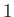and a particle of type. Let the mass and instantaneous velocity of the former particle be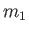and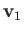, respectively. Likewise, let the mass and instantaneous velocity of the latter particle be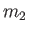and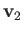, respectively. The velocity of the center of mass is given by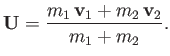(3.10)

Moreover, conservation of momentum implies that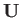is a constant of the motion. The relative velocity is defined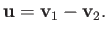(3.11)

We can expressandin terms ofand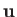as follows: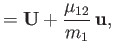(3.12)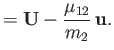(3.13)

Here,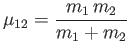(3.14)

is the reduced mass. The total kinetic energy of the system is written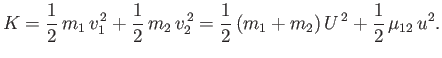(3.15)

Now, the kinetic energy is the same before and after an elastic collision. Hence, given that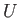is constant, we deduce that the magnitude of the relative velocity,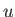, is also the same before and after such a collision. Thus, it is only the direction of the relative velocity vector, rather than its length, that changes during an elastic collision.Next: Boltzmann Collision Operator Up: Collisions Previous: Collision Operator
Richard Fitzpatrick 2016-01-23